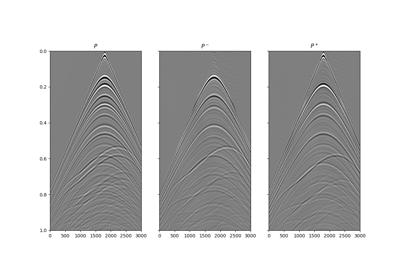# pylops.waveeqprocessing.Deghosting¶

pylops.waveeqprocessing.Deghosting(p, nt, nr, dt, dr, vel, zrec, pd=None, win=None, npad=(11, 11), ntaper=(11, 11), restriction=None, sptransf=None, solver=<function lsqr>, dottest=False, dtype='complex128', **kwargs_solver)[source]

Wavefield deghosting.

Apply seismic wavefield decomposition from single-component (pressure) data. This process is also generally referred to as model-based deghosting.

Parameters: p : np.ndarray Pressure data of of size $$\lbrack n_{r_x}\,(\times n_{r_y}) \times n_t \rbrack$$ (or $$\lbrack n_{r_{x,\text{sub}}}\, (\times n_{r_{y,\text{sub}}}) \times n_t \rbrack$$ in case a restriction operator is provided. Note that $$n_{r_{x,\text{sub}}}$$ (and $$n_{r_{y,\text{sub}}}$$) must agree with the size of the output of this operator) nt : int Number of samples along the time axis nr : Number of samples along the receiver axis (or axes) dt : float Sampling along the time axis dr : Sampling along the receiver array of the separated pressure consituents vel : float Velocity along the receiver array (must be constant) zrec : float Depth of receiver array pd : np.ndarray, optional Direct arrival to be subtracted from p win : np.ndarray, optional Time window to be applied to p to remove the direct arrival (if pd=None) ntaper : float or tuple, optional Number of samples of taper applied to propagator to avoid edge effects npad : float or tuple, optional Number of samples of padding applied to propagator to avoid edge effects angle restriction : pylops.LinearOperator, optional Restriction operator sptransf : pylops.LinearOperator, optional Sparsifying operator solver : float, optional Function handle of solver to be used if kind='inverse' dottest : bool, optional Apply dot-test dtype : str, optional Type of elements in input array. If None, directly inferred from p **kwargs_solver Arbitrary keyword arguments for chosen solver pup : np.ndarray Up-going wavefield pdown : np.ndarray Down-going wavefield

Notes

Up- and down-going components of seismic data $$p^-(x, t)$$ and $$p^+(x, t)$$ can be estimated from single-component data $$p(x, t)$$ using a ghost model.

The basic idea  is that of using a one-way propagator in the f-k domain (also referred to as ghost model) to predict the down-going field from the up-going one (excluded the direct arrival and its source ghost referred here to as $$p_d(x, t)$$):

$p^+ - p_d = e^{-j k_z 2 z_\text{rec}} p^-$

where $$k_z$$ is the vertical wavenumber and $$z_\text{rec}$$ is the depth of the array of receivers

In a matrix form we can thus write the total wavefield as:

$\mathbf{p} - \mathbf{p_d} = (\mathbf{I} + \Phi) \mathbf{p}^-$

where $$\Phi$$ is one-way propagator implemented via the pylops.waveeqprocessing.PhaseShift operator.

  Amundsen, L., 1993, Wavenumber-based filtering of marine point-source data: GEOPHYSICS, 58, 1335–1348.

## Examples using pylops.waveeqprocessing.Deghosting¶13. Deghosting